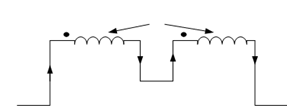## Series Aiding Assignment Help

Assignment Help: >> Inductive Coupling in Series - Series Aiding

Series Aiding:

Figure illustrates two coils coupled in series aiding. Let L1 be the self-inductance of coil1, L2 be the self-inductance of coil 2 and M be the mutual inductance among the two coils.Figure: Inductive Coupling in Series (Flux Aiding)

Let us consider coil 1 first.

Coil 1

Self-induced e.m.f e2  =- L1  (di/dt)

Mutually induced e.m.f e1′ =- M(di/dt) [due to change of current in coil 2]

Coil 2

Let us consider coil 2 now.

Self-induced e.m.f e2  =- L2  (di /dt)

Mutually induced e.m.f e2′ =- M ( di/ dt)  [because of change of current in coil 1].

Thus the total induced e.m.f of the series coupled coils may be written as

e = e1  + e2  + e1′ + e2

or,  e =- L1  (di /dt) - L2 (di /dt) - 2 M(di /dt) =- (L1 + L2  + 2M ) (di /dt )

If L is the equivalent inductance of the coil, then we may write

e =- L (di /dt)

From Eqs. (32) and (33), we get

or, - L(di /dt)   = - (L1 + L2  + 2M ) (di /dt)

or, L = L1  + L2  + 2M#### Assured A++ Grade

Get guaranteed satisfaction & time on delivery in every assignment order you paid with us! We ensure premium quality solution document along with free turntin report!

All rights reserved! Copyrights ©2019-2020 ExpertsMind IT Educational Pvt Ltd